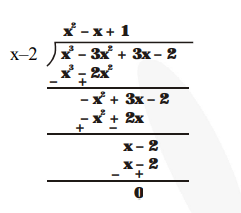# On dividing $x^{3}-3 x^{2}+x+2$ by a polynomial $g(x)$

Question.

On dividing $x^{3}-3 x^{2}+x+2$ by a polynomial $g(x)$, the quotient and remainder were $x$ $-2$ and $-2 x+4$, respectively. Find $g(x)$

Solution:

$\left(x^{3}-3 x^{2}+x+2\right)=g(x) \times(x-2)+(-2 x+4)$

$\Rightarrow x^{3}-3 x^{2}+x+2+2 x+-4=g(x) \times(x-2)$

$\Rightarrow x^{3}-3 x^{2}+3 x-2=g(x) \times(x-2)$

$g(x)=\frac{x^{3}-3 x^{2}+3 x-2}{x-2}$So, $g(x)=x^{2}-x+1$